# Multiplication and Division of Fractions

Students deepen their understanding of fraction multiplication and begin to explore to fraction division (and fractions as division), as well as apply this new understanding to the context of line plots.

Math

Unit 5

## Unit Summary

In Grade 5 Unit 5, students continue their study of fraction operations, deepening their understanding of fraction multiplication from Grade 4 and beginning to explore fraction division.

Students began learning about fractions very early, as described in the Unit 4 Unit Summary. Their exposure to fraction multiplication more specifically began in Grade 4, when they learned to multiply a fraction by a whole number, thinking of this as a whole number of groups of fractional-sized groups. For example, $${4\times {2\over3}}$$ is thought of as 4 groups of 2 thirds.

The unit begins with students developing a new understanding of fractions as division. In the past, they’ve thought of fractions as equal-sized partitions of wholes, but here they develop an understanding of a fraction as an operation itself and represent division problems as fractions (5.NF.3). Students now see that remainders can be interpreted in yet another way, namely divided by the divisor to result in a mixed-number quotient. For example, cutting a 7-foot string into 3 pieces of equal length results in pieces that are $$2\tfrac13$$ feet long. Then, students develop a new understanding of fraction multiplication as fractional parts of a group of a certain size (5.NF.4). For example, they interpret the expression $$\tfrac23\times4$$ as 2 thirds of 4, and thus can be seen as 2 parts of a partition of 4 into 3 equal parts. They also develop general methods to multiply fractions by whole numbers and fractions, including mixed numbers. Throughout this work, students develop an understanding of multiplication as scaling (5.NF.5), “an important opportunity for students to reason abstractly” (MP.2) as the Progressions notes (Progressions for the Common Core State Standards in Mathematics, Number and Operations - Fractions, 3-5, p. 14). Then, students explore division of a unit fraction by a whole number and a whole number by a unit fraction (5.NF.7), preparing students to divide with fractions in all cases in Grade 6 (6.NS.1). Next, students solve myriad word problems, seeing the strategies they used to solve word problems with whole numbers still apply but that special attention should be paid to the whole being discussed (5.NF.6, MP.4), as well as write and solve expressions involving fractions as a way to support the major work (5.OA.1, 5.OA.2). Finally, students make line plots to display a data set of measurements in fractions of a unit and solve problems involving information presented in line plots (5.MD.2), a supporting cluster standard that supports the major work of this and the past unit of using all four operations with fractions (5.NF).

In Unit 6, students will learn to multiply and divide decimals, relying on their understanding of these operations with fractions developed in this unit to do so. In Grade 6, students encounter the remaining cases of fraction division (6.NS.1). “Work with fractions and multiplication is a building block for work with ratios. In Grades 6 and 7, students use their understanding of wholes and parts to reason about ratios of two quantities, making and analyzing tables of equivalent ratios, and graphing pairs from these tables in the coordinate plane. These tables and graphs represent proportional relationships, which students see as functions in Grade 8” (NF Progression, p. 20). Students will further rely on this operational fluency throughout the remainder of their mathematical careers, from fractional coefficients in functions to the connection between irrational numbers and non-repeating decimals.

Pacing: 28 instructional days (25 lessons, 2 flex days, 1 assessment day)

For guidance on adjusting the pacing for the 2021-2022 school year, see our 5th Grade Scope and Sequence Recommended Adjustments.

Fishtank Plus for Math

Unlock features to optimize your prep time, plan engaging lessons, and monitor student progress.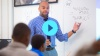## Assessment

This assessment accompanies Unit 5 and should be given on the suggested assessment day or after completing the unit.

## Unit Prep

### Intellectual Prep

#### Intellectual Prep for All Units

• Read and annotate “Unit Summary” and “Essential Understandings” portion of the unit plan.
• Do all the Target Tasks and annotate them with the “Unit Summary” and “Essential Understandings” in mind.
• Take the Post-Unit Assessment.

#### Unit-Specific Intellectual Prep

• While students learned the two meanings of division in Grade 3, they are still important when working with 5.NF.7. To read about the two interpretations of fraction division, read the following blog post by Bill McCallum and Kristin Umland, Fraction division part 2: Two interpretations of division. This is part 2 of 4 of blog posts on fraction division, and the other parts are optional.
• Read the following table that includes models used in this unit.
 Set model Example: Use a set model to solve $$\frac{1}{4}\times12$$.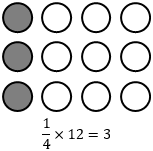Area model Example: Use an area model to solve $$\frac{2}{5}\times\frac{3}{4}$$.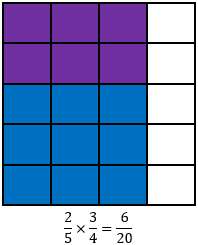Tape diagram Example: Use a tape diagram to solve $$\frac{1}{2}\div3$$.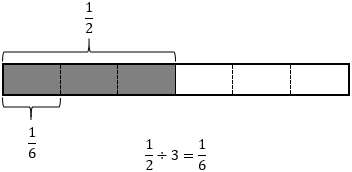Number line Example: Use a number line to solve $$2\div\frac{1}{4}$$.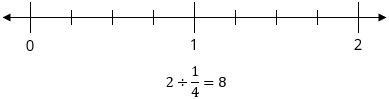Line plot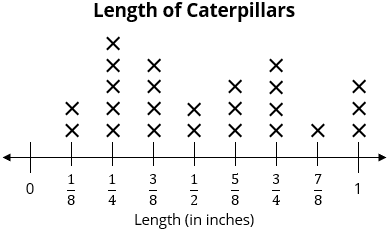### Essential Understandings

• Properties of operations still hold even when the terms are fractions, and they can be used to simplify a computation.
• Fractions can be interpreted as division. As a result, a remainder in a division problem can be divided by the divisor and represented as a fraction.
• Multiplication of a fraction and a whole number can be thought of in two ways. For example, $${4\times {{{2\over3}}}}$$ can be seen as 4 groups of $${{2\over3}}$$, an interpretation students relied on in Grade 4. But, $$4\times{{{2\over3}}}$$ can also be seen as 2 parts of a partition of 4 into 3 equal parts, a new interpretation that students will encounter in this unit.
• Multiplication can result in a smaller or equivalent product. Similarly, division can result in a larger or equivalent quotient.

## Unit Practice

Word Problems and Fluency Activities

Access daily word problem practice and our content-aligned fluency activities created to help students strengthen their application and fluency skills.## Lesson Map

Topic A: Fractions as Division

Topic B: Multiplying a Fraction by a Whole Number

Topic C: Multiplying a Fraction by a Fraction

Topic D: Multiplying with Mixed Numbers

Topic E: Dividing with Fractions

Topic F: Fraction Expressions and Real-World Problems

Topic G: Line Plots

## Common Core Standards

Key

Major Cluster

Supporting Cluster

### Core Standards

#### Measurement and Data

• 5.MD.B.2 — Make a line plot to display a data set of measurements in fractions of a unit (1/2, 1/4, 1/8). Use operations on fractions for this grade to solve problems involving information presented in line plots. For example, given different measurements of liquid in identical beakers, find the amount of liquid each beaker would contain if the total amount in all the beakers were redistributed equally.

#### Number and Operations—Fractions

• 5.NF.B.3 — Interpret a fraction as division of the numerator by the denominator (a/b = a ÷ b). Solve word problems involving division of whole numbers leading to answers in the form of fractions or mixed numbers, e.g., by using visual fraction models or equations to represent the problem. For example, interpret 3/4 as the result of dividing 3 by 4, noting that 3/4 multiplied by 4 equals 3, and that when 3 wholes are shared equally among 4 people each person has a share of size 3/4. If 9 people want to share a 50-pound sack of rice equally by weight, how many pounds of rice should each person get? Between what two whole numbers does your answer lie?
• 5.NF.B.4 — Apply and extend previous understandings of multiplication to multiply a fraction or whole number by a fraction.
• 5.NF.B.4.A — Interpret the product (a/b) × q as a parts of a partition of q into b equal parts; equivalently, as the result of a sequence of operations a × q ÷ b. For example, use a visual fraction model to show (2/3) × 4 = 8/3, and create a story context for this equation. Do the same with (2/3) × (4/5) = 8/15. (In general, (a/b) × (c/d) = ac/bd.)
• 5.NF.B.4.B — Find the area of a rectangle with fractional side lengths by tiling it with unit squares of the appropriate unit fraction side lengths, and show that the area is the same as would be found by multiplying the side lengths. Multiply fractional side lengths to find areas of rectangles, and represent fraction products as rectangular areas.
• 5.NF.B.5 — Interpret multiplication as scaling (resizing), by:
• 5.NF.B.5.A — Comparing the size of a product to the size of one factor on the basis of the size of the other factor, without performing the indicated multiplication.
• 5.NF.B.5.B — Explaining why multiplying a given number by a fraction greater than 1 results in a product greater than the given number (recognizing multiplication by whole numbers greater than 1 as a familiar case); explaining why multiplying a given number by a fraction less than 1 results in a product smaller than the given number; and relating the principle of fraction equivalence a/b = (n×a)/(n×b) to the effect of multiplying a/b by 1.
• 5.NF.B.6 — Solve real world problems involving multiplication of fractions and mixed numbers, e.g., by using visual fraction models or equations to represent the problem.
• 5.NF.B.7 — Apply and extend previous understandings of division to divide unit fractions by whole numbers and whole numbers by unit fractions. Students able to multiply fractions in general can develop strategies to divide fractions in general, by reasoning about the relationship between multiplication and division. But division of a fraction by a fraction is not a requirement at this grade
• 5.NF.B.7.A — Interpret division of a unit fraction by a non-zero whole number, and compute such quotients. For example, create a story context for (1/3) ÷ 4, and use a visual fraction model to show the quotient. Use the relationship between multiplication and division to explain that (1/3) ÷ 4 = 1/12 because (1/12) × 4 = 1/3.
• 5.NF.B.7.B — Interpret division of a whole number by a unit fraction, and compute such quotients. For example, create a story context for 4 ÷ (1/5), and use a visual fraction model to show the quotient. Use the relationship between multiplication and division to explain that 4 ÷ (1/5) = 20 because 20 × (1/5) = 4.
• 5.NF.B.7.C — Solve real world problems involving division of unit fractions by non-zero whole numbers and division of whole numbers by unit fractions, e.g., by using visual fraction models and equations to represent the problem. For example, how much chocolate will each person get if 3 people share 1/2 lb of chocolate equally? How many 1/3-cup servings are in 2 cups of raisins?

#### Operations and Algebraic Thinking

• 5.OA.A.1 — Use parentheses, brackets, or braces in numerical expressions, and evaluate expressions with these symbols.
• 5.OA.A.2 — Write simple expressions that record calculations with numbers, and interpret numerical expressions without evaluating them. For example, express the calculation "add 8 and 7, then multiply by 2" as 2 × (8 + 7). Recognize that 3 × (18932 + 921) is three times as large as 18932 + 921, without having to calculate the indicated sum or product.

• 3.MD.C.7
• 4.MD.B.4

• 3.NF.A.1
• 4.NF.A.1
• 4.NF.B.3
• 4.NF.B.4
• 5.NF.A.1
• 5.NF.A.2

• 3.OA.A.1
• 3.OA.A.2
• 3.OA.B.6
• 3.OA.D.8
• 4.OA.A.1
• 4.OA.A.2
• 4.OA.A.3

• 5.NBT.B.7

• 6.RP.A.1
• 6.RP.A.2

• 6.SP.A.1
• 6.SP.A.2
• 6.SP.A.3
• 6.SP.B.4

• 6.NS.A.1
• 6.NS.B.4
• 7.NS.A.2

### Standards for Mathematical Practice

• CCSS.MATH.PRACTICE.MP1 — Make sense of problems and persevere in solving them.

• CCSS.MATH.PRACTICE.MP2 — Reason abstractly and quantitatively.

• CCSS.MATH.PRACTICE.MP3 — Construct viable arguments and critique the reasoning of others.

• CCSS.MATH.PRACTICE.MP4 — Model with mathematics.

• CCSS.MATH.PRACTICE.MP5 — Use appropriate tools strategically.

• CCSS.MATH.PRACTICE.MP6 — Attend to precision.

• CCSS.MATH.PRACTICE.MP7 — Look for and make use of structure.

• CCSS.MATH.PRACTICE.MP8 — Look for and express regularity in repeated reasoning.

Unit 4